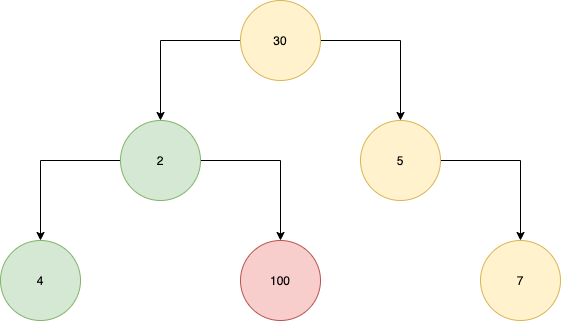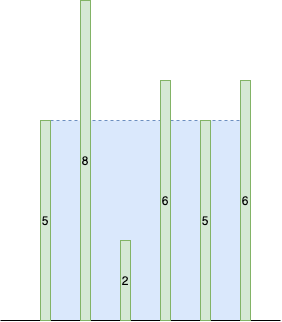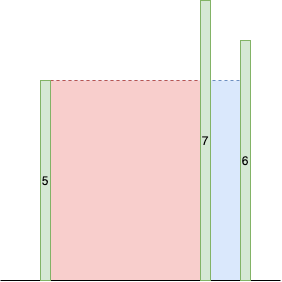# Audience

This article is aimed at engineers with a reasonably good understanding of programming, data structures and algorithms. It is mainly based in Java, so an understanding of the language is very useful. However, as long as you appreciate its core concepts you should be fine.

# Argument

Greedy algorithms are used in optimisation problems and function by making the optimal choice at each step. The combination of these choices should then create the best solution to the overall task!

An important point to note is that we never go back to reconsider a previous solution. Once we have made a choice we need to stick with it.

They function by setting a rule for which we add to a solution at each step. For example, say we are traversing the below tree.Traversing the tree using a greedy algorithm

The greedy algorithm we have defined takes the largest number at each level of the tree in a bid to find the path with the maximum sum.

However, we can see that it fails. By taking the largest value at the second layer we ignore that in later layers we may have a larger value! This is an excellent demonstration of when greedy algorithms are not suitable. In these cases we may be better served by dynamic programming.

So when do we pick a greedy algorithm? There are two properties we look for.

• Greedy choice property: The overall optimal solution can be found by using the optimal choice at each step.
• Optimal substructure: The overall optimal solution contains the optimal solutions to the sub-problems.

Although this may seem a little abstract, let’s clarify with some examples. First let’s look at Djikstra, then move to some LeetCode problems.

## Djikstra

Pretend we have the below graph, we are starting at `A` and trying to get to `E`.

What we are looking to do is to generate a table similar to the below.

Our table has three columns: the vertex we are looking to find, the shortest distance from our original vertex, and the previous vertex in our shortest path.

It’s really important to understand that the shortest route to a node `v` is equal to the shortest route to the node connected to `v`, combined with the smallest distance to `v` itself. For example the shortest route to get to `D` is equal to the minimum of:

1. Shortest route to get to `B` plus the `B-D` edge weight
2. Shortest route to get to `C` plus the `B-C` edge weight.

This is as `B` and `C` are the only two nodes connected to `D`, except for `E` which is our target!

Hopefully this will become clearer with an example. For the algorithm we need two lists: visited and unvisited. We also initiate our table as below.

We repeat the below steps to carry out Djikstra:

1. Visit the node with the smallest distance from the start node.
2. For the current node examine its unvisited neighbours.
3. Calculate the distance of each neighbour to the start node where the path goes through the current node (this is the same as the current node’s minimum distance to the start node + the edge weight to the neighbour node).
4. If the calculated distance is less than the known distance, update the shortest distance in the neighbour node.
5. If we have updated the nodes shortest distance update the previous vertex of the updated node. This is now as we will step back through our current node to find the shortest route!
6. Add current node to the list of visited nodes.

Let’s see how that might work in our example.

We start at node `A`(1), and examine `B` and `C` (2). We calculate the distance to the start node (3), `B`‘s distance is `2`, `C`‘s distance is `7`. We update both of these in the table as they are less than infinite (4), and set the previous node to `A` (5). We then add `A` to our list of visited nodes (6). We start again from `B` (closest node).

So where is the greedy component? We always choose the closest node to go to next (optimal choice at that step). Once we’ve done so we know we have the shortest path to that node. Doing this for all the nodes gives us the result (overall optimal solution).

## Containers and Water

This example is taken from the LeetCode problem here. Have a look at the problem description, then come back and we can explore it.

The idea is that we have a number of walls we can use, and we must create a container to hold the most water using the walls and the floor.

For example, let’s say we have an array `[5, 8, 2, 6, 5, 8]`. Each value in the array represents the wall we can put down at that position.An example of our walls and our solution

How does this lend itself to our issue? Well let’s think about how we might solve the problem.

A brute force approach would run through all possible combinations of walls: `(0, 1), (0, 2), ... ,(1, 2), (1, 3), ... (2, 3), (2, 4), ...`. This would get us there, but would run in `O(n^2)` time. Tricky.

There is a trick though. Let’s think about the case where we put down a wall at position 0, and a wall at position 5. I’ve also included another potential wall at 4 for clarity.Wall at zero, wall at five

If the wall at zero is smaller than the wall at five then we know this is the maximum height we can achieve with a wall starting at zero, as the `x` value will always decrease, making a smaller area (indicated in red).

If the wall at zero is larger than the wall at five we could potentially make a larger area by moving the wall at five inwards.

This way we come up with a rule. We have two pointers, one at the beginning, one at the end. If the wall at the pointer at the beginning is larger than the wall at the pointer at the end we move the pointer at the end in, to make a potentially larger area. Otherwise we have reached the maximum for the beginner pointer and move it in.

We can formalise this in the solution below.

So how is this greedy? We always choose walls larger or equal to previously (optimal choice at that step). Once we’ve done so we know we can calculate the largest area for the previously considered set of walls. Doing this for all the walls gives us the result (overall optimal solution).

## Interval Scheduling

The final type of problem we will explore is interval scheduling. In this scenario we have a number of tasks with a start and end time, and we need to schedule the maximum number of them.

Our greedy approach requires us to define candidate jobs. These are jobs with no overlaps and which have not already been scheduled. The overall idea is to order the jobs by their end time, then to pick the first one and schedule it. From there we pick the next job which does not overlap with the earliest end time.

This approach is `O(n log(n))` in complexity, as we must first order our intervals by their end times (`O(n log(n)`) then go through each of the intervals and see if we can schedule them (`O(n)`).

We can write up a solution as below. We are assuming the intervals come in in an array, where `intervals[i]` is equal to a nested array `interval` where `interval` is the start and `interval` is the end.

This is greedy as by choosing the earliest possible finishing interval we leave the most time to fill with other intervals (optimal choice at that step). Doing this for all the intervals gives us the result (overall optimal solution).

# Conclusion

In conclusion, we have covered greedy algorithms, their definition and explored a couple of examples.

--

--